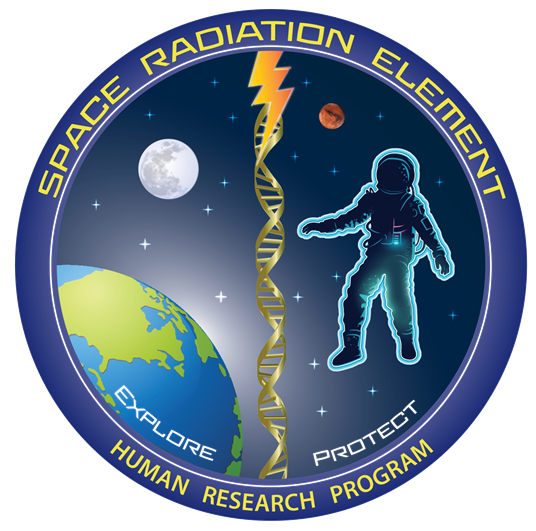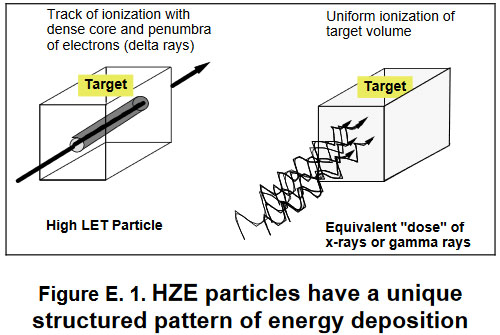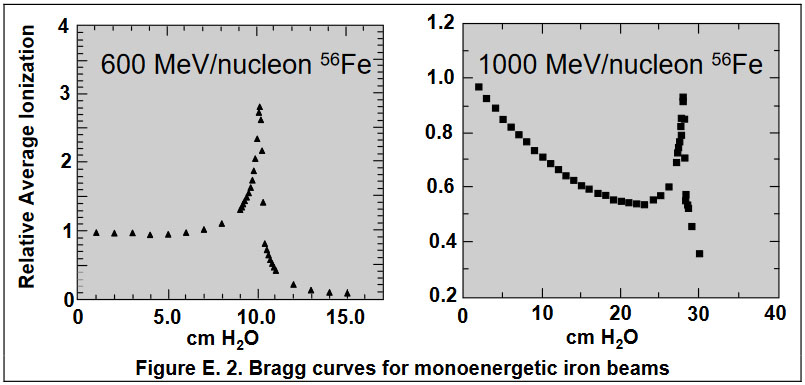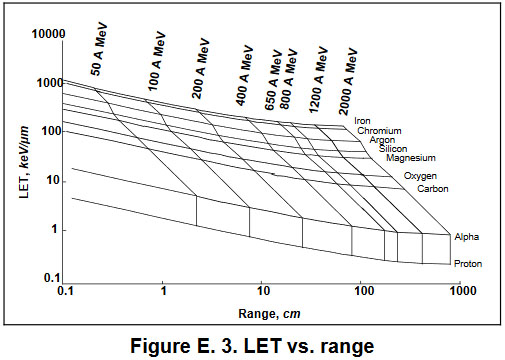Policies and Procedures

Instructions to Authors

Annual Report

NSRL

## Interactions of Radiation with Matter

Walter Schimmerling, Ph.D.
2/7/2011

Basic Concepts

The high-energy charged particles constituting galactic cosmic rays are nuclei of all the elements, accelerated to high energies, probably in supernova explosions within our galaxy. While nuclei of elements are also ejected by solar disturbances, the solar radiation of significance for radiation protection consists mainly of protons, which are the nuclei of hydrogen atoms. These nuclei are not surrounded by electrons, as would be the case for neutral atoms of elements found on Earth. They consist of Z protons and N neutrons. The chemical properties of an element are defined by the number of protons, Z, also known as its atomic number, and by its atomic weight. In the absence of electrons, the atomic weight is A (=N+Z), although even in the fully neutral atom the Z electrons surrounding the nucleus contribute only a negligible amount to the total mass.

The neutrons and protons are generically called "nucleons" to indicate that they share a major property: the response to the nuclear forces that keep the repulsion between the Z electrically charged protons from disintegrating the nucleus. A "standard nucleon", or atomic mass unit (amu) is defined as the average mass per particle of a 12C nucleus (containing exactly 6 protons and 6 neutrons). This corresponds to approximately 1.7X10-27 kg. According to Einstein's relation, E=mc2 (where E is the relativistic total energy, c is the speed of light, 3X108 m/sec, or 186,000 miles/sec and m is the mass), if an amu were entirely converted into energy it would be equivalent to approximately 931 MeV, which is the unit in which the masses are commonly described. For comparison, the equivalent amount of energy contained in an electron mass, 0.51 MeV, is almost 2000 smaller, with notable effect on their interaction with matter.

The high energy of these nuclei means that they are moving so fast that their velocity is measured as a fraction of the speed of light, c. The nucleons inside the nucleus all move at the same velocity, since they are traveling as a group. The speeding nuclei will interact with the materials they penetrate, and will manifest different properties depending on the type of interaction.

Interactions with atomic electrons are due to the electrical interaction between the (negative) charge of the electrons of elements in the material and the (positive) charge of the protons in the projectile. These charges are sensed by each of the participants over large distances, where the protons in the incident nucleus appear as a single cluster with charge Z, moving at the average velocity of the nucleons. These interactions are entirely similar to friction, and cause the fast, high energy particles to slow down. When the energy transferred to atomic electrons is large enough, one or more electrons may be removed from the atom altogether, leading to a disruption of the chemical bonds that link atoms and molecules. Evidently, when the disrupted molecules are inside a living cell, serious damage is likely to occur.

Every once in a while, the track of a nucleus penetrating through materials will come close to the nuclei of atoms in the material. When this happens, the target nuclei, the projectile nuclei, or both may break up and energetic parts of nuclei will then fly off and continue to interact with the material. These secondary radiations may be gamma rays, neutrons, protons, nucleon clusters, or subatomic particles, such as µ-mesons, created in the collision. Since these collisions occur at close quarters, it will be sensitive to the position and velocity of individual interacting nucleons from both the projectile and the target.

The energy available for each collision of nucleons will be the energy per nucleon, and not the kinetic energy of the whole nucleus, unless the nucleus interacts as a coherent unit. For this reason, the energy of HZE particles is commonly described in terms of energy/nucleon. For example, in an HZE nucleus consisting of A nucleons moving at 80% of the speed of light, the energy per nucleon is 620 MeV/nucleon, which can also be written as 620A MeV.

# Energy Loss

Heavy charged particles lose energy in matter in a manner entirely different from other types of radiation. A proton or HZE particle suffers a very large number of energy losses interacting with atomic electrons in materials. Each of these losses is generally very small from the point of view of the incident particle, but may be quite large from the perspective of the electrons in the path. Especially while the particle is still going at high speed, the energy may be sufficient to detach the electron from its atom (i.e., ionize the atom) while imparting only small deviations to the trajectory of the incident particle. The emerging electron may have sufficient energy to ionize other electrons in its turn,and a cloud of electrons of very many energies will be generated as a track around the particle trajectory. The pattern of energy loss of a proton or HZE particle will thus be characterized by a dense track of ionizations and atomic excitations, along a straight line corresponding to the particle trajectory. The high density of energy loss per unit path length is described as the Linear Energy Transfer (LET). These facts are summarized in the left sketch of Figure E. 1.

In contrast, electrons, even at high energies, are so light that most collisions with other electrons will result in a significant deviation from their path. Thus, the path of an electron in matter consists of a very large number of changes of direction. Radiation that does not consist of charged particles, such as x-rays, gamma rays and neutrons, in general will only ionize atoms in the material indirectly. X-ray and gamma ray photons can be absorbed by electrons and atoms to yield energetic electrons (and, sometimes, secondary photons), and neutrons generate charged particles in nuclear interactions. In either case, the probability of an energy deposition event is uniform throughout the target volume, as shown in the right-hand panel of Figure E. 1.

In the case of indirectly ionizing radiation, photons or neutrons are removed from the incident number as a function of depth, with a constant probability per unit path length (described by a cross section) leading to an exponential pattern of energy deposition as a function of depth. In contrast, heavy charged particles are characterized by a well-defined range. This is the average penetration distance. It depends on the charge, the mass and the velocity of the incident particle and on the material properties. Of these, the most important is the electron density in the traversed medium, which is related to the composition of the elements out of which the material is made.The number of energy loss collisions suffered by heavy charged particles is very large, the statistical fluctuations will be relatively small, and all particles of a given kind, with the same initial energy, will stop very close to each other when all their energy is spent. Furthermore, while particles are still proceeding at high velocity, relatively little time is spent close to atoms, where the attraction to atomic electrons is strongest; thus, energy losses are relatively small until the particle has slowed down considerably, near the end of its range. These facts are summarized in the plot of average relative ionization of a monoenergetic beam of particles, as a function of depth, shown in Figure E. 2. Such a plot is known as a "Bragg curve", after its discoverer. In Figure E. 2, data are shown for two beams of iron particles incident on water, one incident at 600 MeV/nucleon and one incident at 1000 MeV/nucleon. The data were obtained using the Brookhaven National Laboratory AGS accelerator. The range of the 600 MeV/nucleon iron beam is approximately 10 cm of water, whereas at 1000 MeV/nucleon the range is approximately 27 cm.

The theory of energy loss of charged particles in matter is well known. Given the charge and mass of a particle and the element composition of the target material, there is a relation between the energy loss per unit path length, the rangeand the energy per nucleon (i.e., the velocity) of the particle, such that, given any two, the third can be calculated. A sample of such calculations has been plotted in Figure E. 3, for the nine most important components of space radiation, in the energy region of interest.

# Nuclear Interactions

The curves in Figure E. 3 assume that the particles suffer no nuclear interactions. In the case of relatively low-energy beams, this assumption is a good approximation. However, in the case of high energy beams, such as the 1000 MeV/nucleon beam shown in the right-hand Bragg curve of Figure E. 2, this is no longer true. The range of such an energetic particle is large enough that loss of particles due to nuclear interactions is significant. This accounts for the exponential decrease in the Bragg curve. However, even for the lower energy beam there is a significant component of ionization due to nuclear interaction products, and almost half the beam at the Bragg peak consists of secondary particles produced in nuclear interaction.

A significant fraction of the radiation flux traversing spacecraft components and in the bodies of personnel will undergo nuclear reactions. Nuclear interactions of charged particles are characterized by the probability of observing a given reaction outcome, per incident particle fluence, per reaction event. This is the cross section for the event, s; it has the dimensions of an area presented to the incident particles. Commensurate with the size of the target nuclei, nuclear cross sections are usually given in units of barns (1 b=10-24 cm2). Typical nuclear reaction cross sections are given in millibarn (mb); by comparison, cross sections for atomic interactions are a million times larger. Cross sections can be a differential of energy, angle of the emerging products, multiplicity of observed particles, and other factors.

Nuclear interactions of the heavy component of GCR are usually classified into three categories: projectile fragmentation, target fragmentation and central collisions. Seen from their respective rest frames, the products of projectile and target fragmentation are emitted with equal probability in all directions (i.e., are isotropic). However, in the (target) frame of reference of the shielded observer, the kinematics of relativistic particles are such that the projectile fragments will be sharply peaked forward in the direction of projectile incidence. Central collisions involve the overlapping region of the colliding nuclei, where multiple collisions and regroupings of the participating nucleons lead to final products that can be emitted into much larger angles. A significant number of GCR interactions result in more than one high-energy particle emerging from the reaction. The multiplicity of GCR reaction products is related to the extent to which the collision is violent enough to break up the reacting nuclei or parts of them.

In order to characterize the radiation field to which personnel in space may be exposed, it is thus necessary to know the energy spectrum, the angular distribution, and the multiplicity of each type of secondary particle. In the cases of interest here, where these reactions can take place anywhere in a thick absorber, it is necessary to know these quantities as a function of particle energy not only for all particles incident upon a thickness of material, but for all the particles produced inside the material as well.

The theory of nuclear reactions of HZE particles is much less developed than other parts of high-energy nuclear physics. In part this is due to the fact that high-energy heavy particle beams have only become available relatively recently; in part, it is due to the fact that the number of possible outcomes in any given nuclear reaction is enormously larger than is usual for lower energies or simpler protons and neutrons. To some extent, the nuclear reactions between HZE particles, at very high energies, are also a field where new ideas of physics are being developed, such as the search for a quark-gluon plasma, for which the new Relativistic Heavy Ion Collider (RHIC) at Brookhaven is intended.